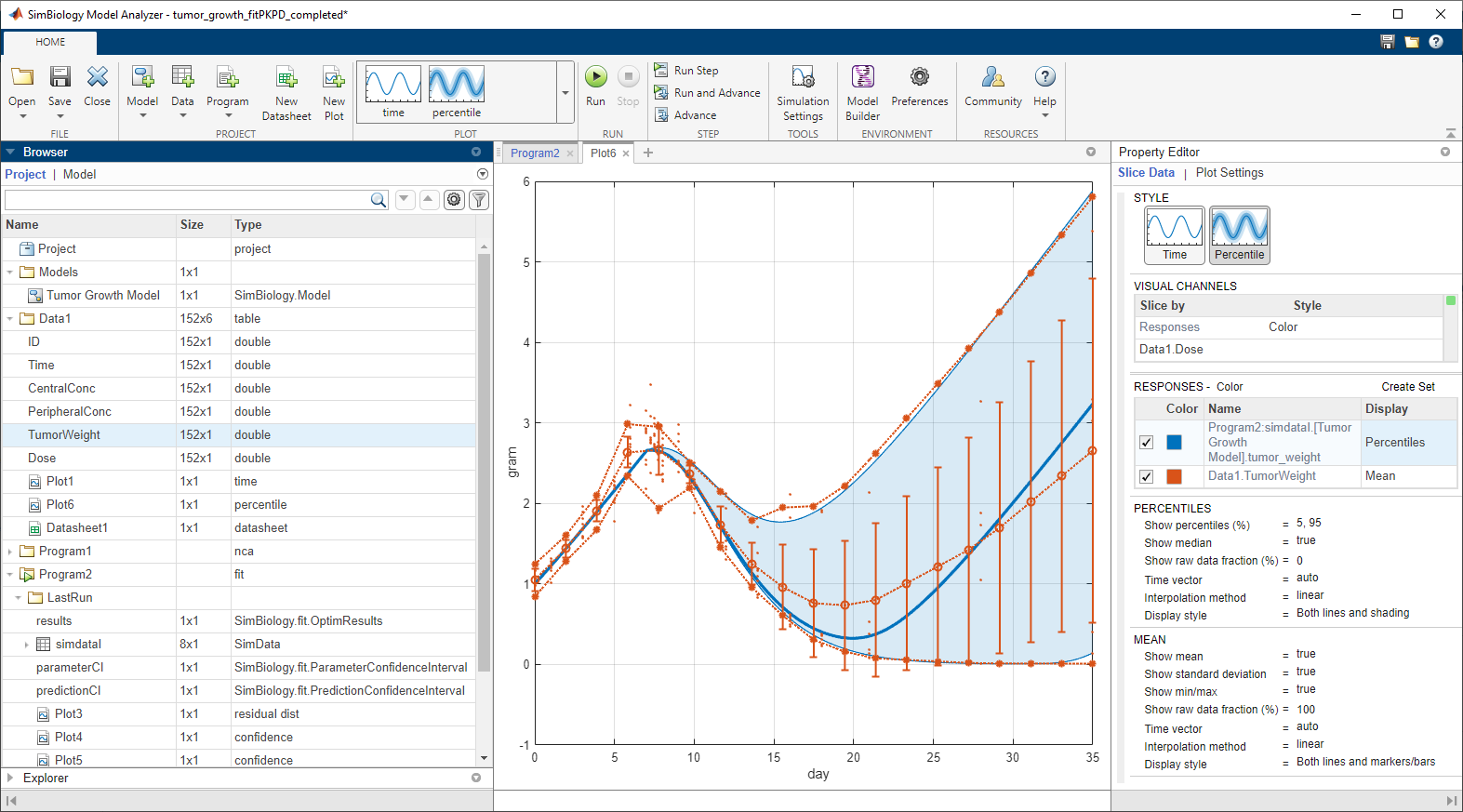Documentation

# SimBiology Model Analyzer

Analyze PK/PD and mechanistic systems biology models

## Description

The SimBiology Model Analyzer app lets you analyze models of dynamic systems such as metabolic networks, signaling pathways, quantitative systems pharmacology (QSP), and pharamacokinetic/pharmacodynamic (PK/PD) of drugs. It provides several analysis methods for your models and various plots to visualize the results.

Using the app, you can:

• Simulate a model to see its dynamic behavior over time.

• Explore biological variability by simulating alternate scenarios such as virtual patients.

• Estimate model parameters using nonlinear regression and nonlinear mixed-effects methods.

• Perform parameter scans and sensitivity analysis to investigate the influence of model parameters and initial conditions on model behavior.

• Specify units and let the app automatically converts the matching physical quantities to one consistent unit system.

• Explore various dosing regimens.

• Perform noncompartmental analysis (NCA) to compute PK parameters of a drug from its PK data.

• View analysis results in various plots.

### Available Plots

The app lets you visualize the analysis results using various plots including:

• Time course of model quantities

• Sensitivity matrix

• Overlay of estimated results on experimental data

• Fit quality measure plots such as confidence intervals, residuals, residual distribution, actual-versus-predicted, and box plot

• Scatter plot matrix## Open the SimBiology Model Analyzer App

• MATLAB® toolstrip: On the Apps tab, under Computational Biology, click the app icon.

• MATLAB command prompt: Enter `simBiologyModelAnalyzer`.

## Programmatic Use

expand all

`simBiologyModelAnalyzer` opens the SimBiology Model Analyzer app.

#### Introduced in R2019b

##### SupportGet trial now